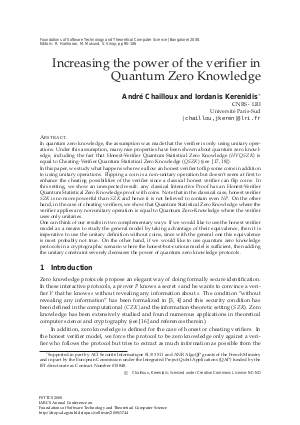Document# Increasing the power of the verifier in Quantum Zero Knowledge

### Authors Andre Chailloux, Iordanis Kerenidis## File

LIPIcs.FSTTCS.2008.1744.pdf
• Filesize: 418 kB
• 12 pages

## Cite As

Andre Chailloux and Iordanis Kerenidis. Increasing the power of the verifier in Quantum Zero Knowledge. In IARCS Annual Conference on Foundations of Software Technology and Theoretical Computer Science. Leibniz International Proceedings in Informatics (LIPIcs), Volume 2, pp. 95-106, Schloss Dagstuhl - Leibniz-Zentrum für Informatik (2008)
https://doi.org/10.4230/LIPIcs.FSTTCS.2008.1744

## Abstract

In quantum zero knowledge, the assumption was made that the verifier is only using unitary operations. Under this assumption, many nice properties have been shown about quantum zero knowledge, including the fact that Honest-Verifier Quantum Statistical Zero Knowledge (\$HVQSZK\$) is equal to Cheating-Verifier Quantum Statistical Zero Knowledge (\$QSZK\$) (see ~\cite{Wat02,Wat06}). In this paper, we study what happens when we allow an honest verifier to flip some coins in addition to using unitary operations. Flipping a coin is a non-unitary operation but doesn\'t seem at first to enhance the cheating possibilities of the verifier since a classical honest verifier can flip coins. In this setting, we show an unexpected result: any classical Interactive Proof has an Honest-Verifier Quantum Statistical Zero Knowledge proof with coins. Note that in the classical case, honest verifier \$SZK\$ is no more powerful than \$SZK\$ and hence it is not believed to contain even \$NP\$. On the other hand, in the case of cheating verifiers, we show that Quantum Statistical Zero Knowledge where the verifier applies any non-unitary operation is equal to Quantum Zero-Knowledge where the verifier uses only unitaries. One can think of our results in two complementary ways. If we would like to use the honest verifier model as a means to study the general model by taking advantage of their equivalence, then it is imperative to use the unitary definition without coins, since with the general one this equivalence is most probably not true. On the other hand, if we would like to use quantum zero knowledge protocols in a cryptographic scenario where the honest-but-curious model is sufficient, then adding the unitary constraint severely decreases the power of quantum zero knowledge protocols.
##### Keywords
• Quantum cryptography
• zero-knowledge protocols
• honest-verifier
• quantum semi-honest model
• hiddenquantum cryptography
• zero-knowledge protocols
• honest-verifier
• quantum semi-honest model
• hidden-bits

## Metrics

• Access Statistics
• Total Accesses (updated on a weekly basis)
0